# How to Calculate and Solve for r.p.m of Driven Pulley | Pulley Size Calculations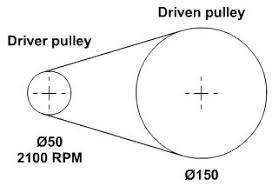The image above represents r.p.m of driven pulley.

To compute for r.p.m of driven pulley, three essential parameters are needed and these parameters are Diameter of Driven Pulley (Db), r.p.m. of Driver Pulley (ra) and Diameter of Driver Pulley (Db).

The formula for calculating r.p.m of driven pulley:

rb = (Da x ra/Db

Where:

rb = r.p.m. of Driven Pulley
Da = Diameter of Driver Pulley
ra = r.p.m. of Driver Pulley
Db = Diameter of Driven Pulley

Let’s solve an example;
Given that the diameter of driver pulley is 10, the r.p.m of driver pulley is 4 and the diameter of driven pulley is 6. Find the r.p.m of driven pulley?

This implies that;

Da = Diameter of Driver Pulley = 10
ra = r.p.m. of Driver Pulley = 4
Db = Diameter of Driven Pulley = 6

rb = (Da x ra/Db
rb = (10 x 4)/6
rb = 40/6
rb = 6.67

Therefore, the r.p.m of driven pulley is 6.67 Hz.

Nickzom Calculator – The Calculator Encyclopedia is capable of calculating the r.p.m of driven pulley.

To get the answer and workings of the r.p.m of driven pulley using the Nickzom Calculator – The Calculator Encyclopedia. First, you need to obtain the app.

You can get this app via any of these means:

To get access to the professional version via web, you need to register and subscribe for NGN 2,000 per annum to have utter access to all functionalities.
You can also try the demo version via https://www.nickzom.org/calculator

Apple (Paid) – https://itunes.apple.com/us/app/nickzom-calculator/id1331162702?mt=8
Once, you have obtained the calculator encyclopedia app, proceed to the Calculator Map, then click on Materials and Metallurgical under Engineering.Now, Click on Ball Mill Sizing under Materials and Metallurgical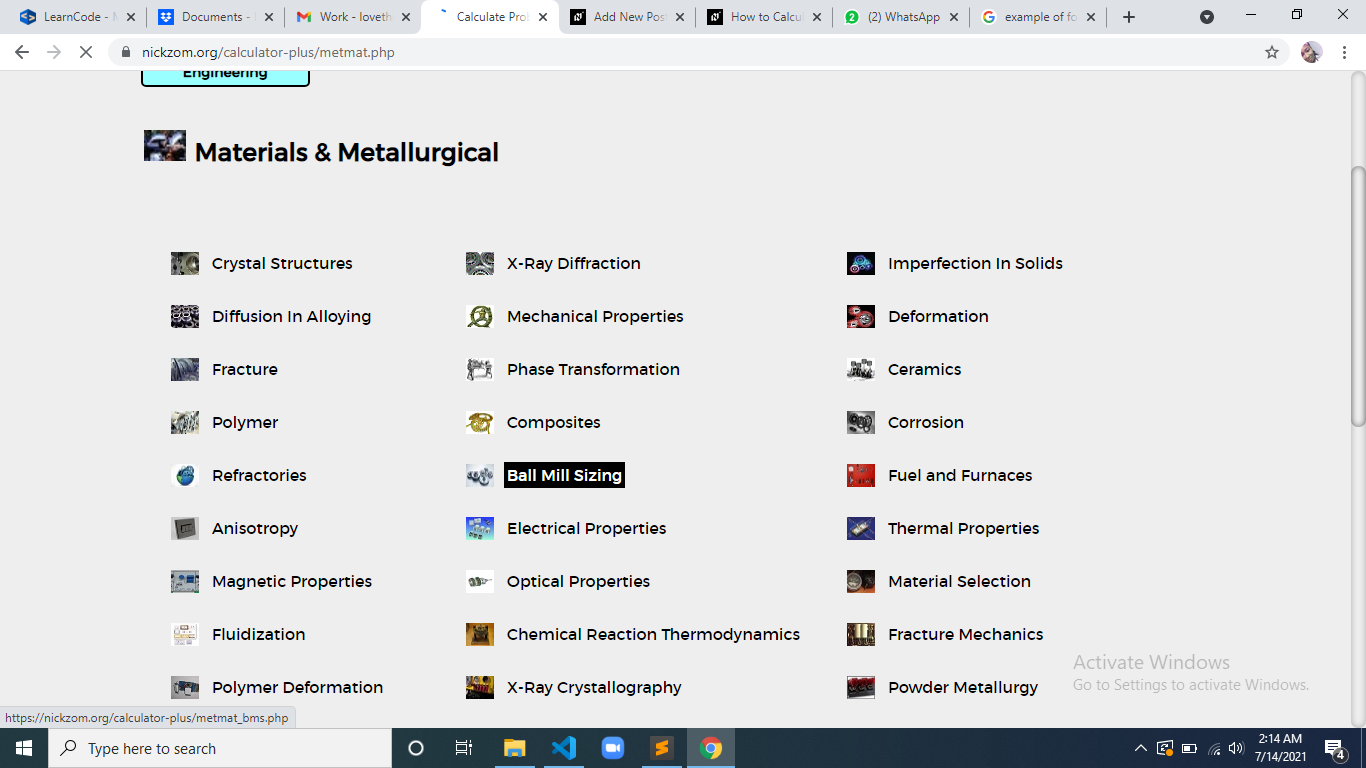Now, Click on Pulley Size under Ball Mill Sizing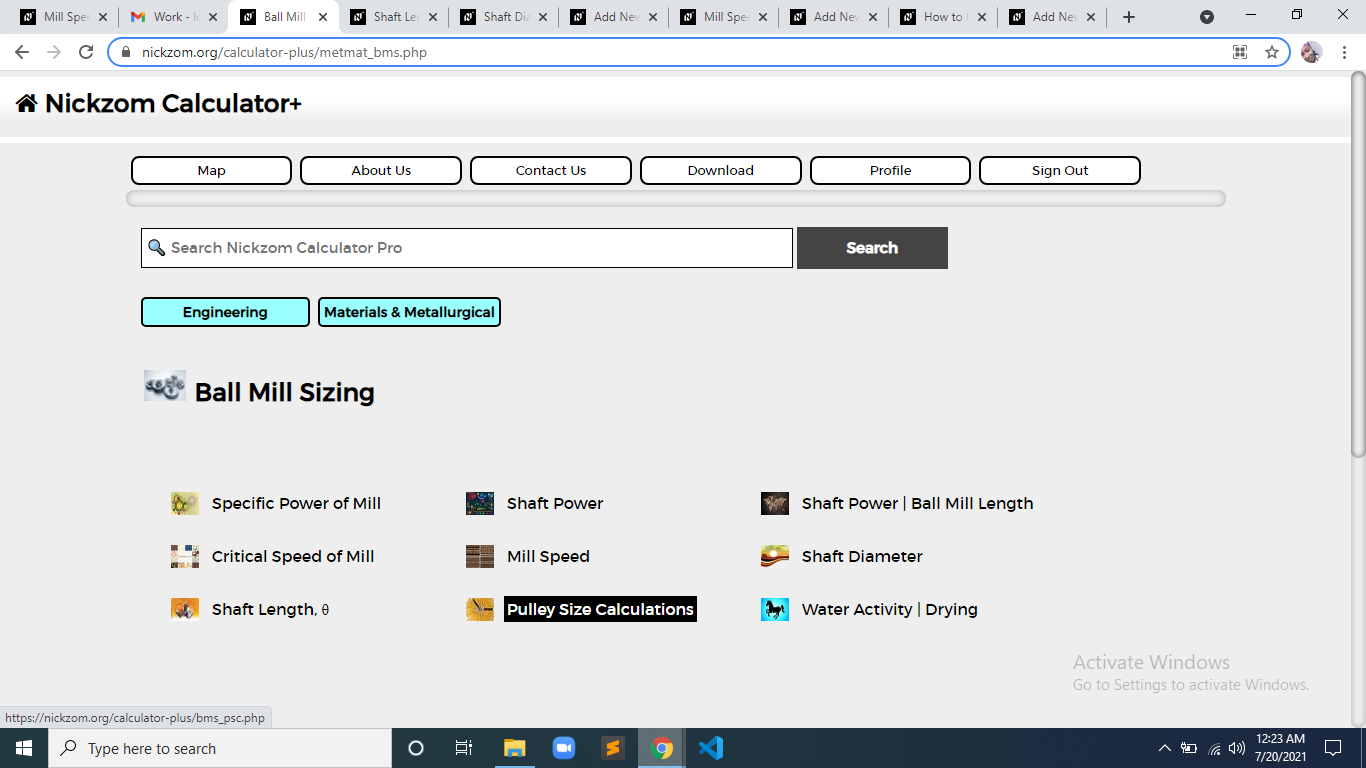Now, Click on r.p.m of Driven Pulley under Pulley Size Calculations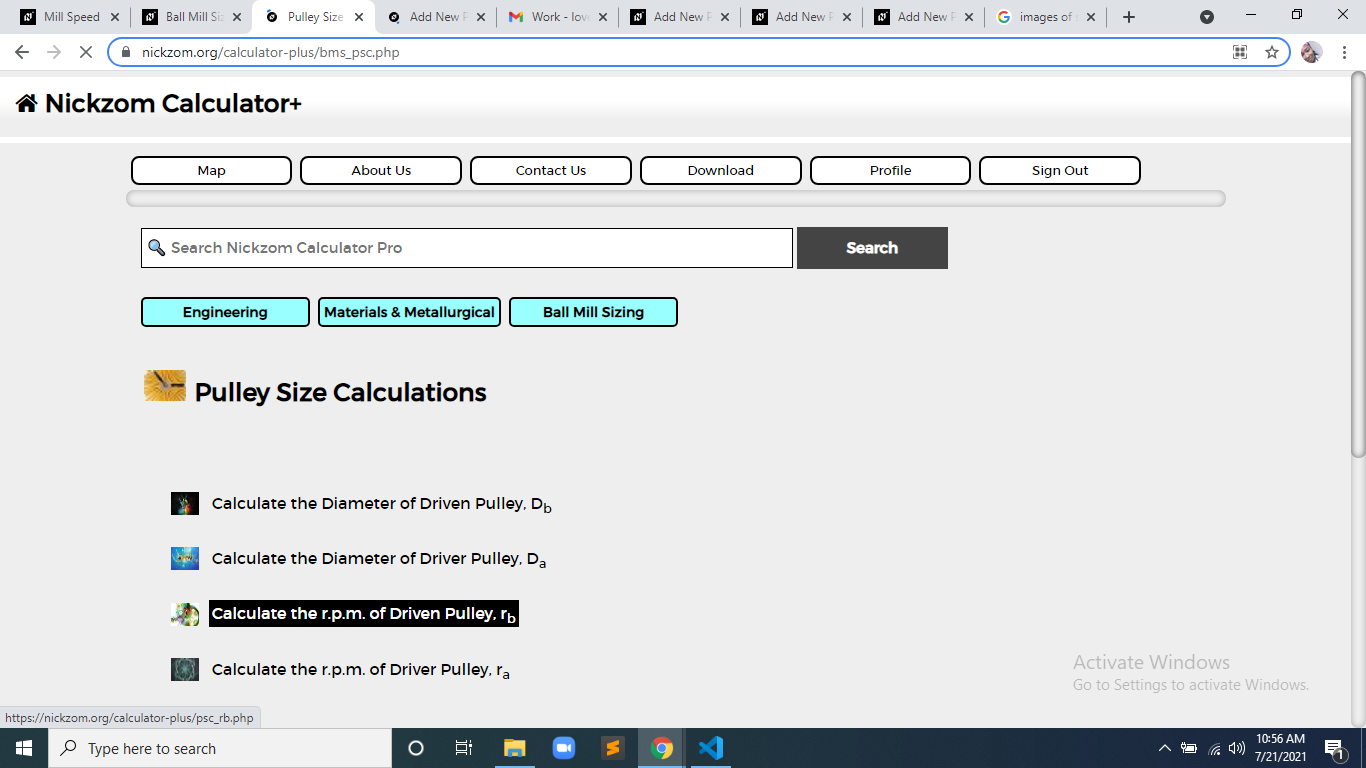The screenshot below displays the page or activity to enter your values, to get the answer for the r.p.m of driven pulley according to the respective parameters which is the Diameter of Driver Pulley (Da), r.p.m. of Driver Pulley (ra) and Diameter of Driven Pulley (Db).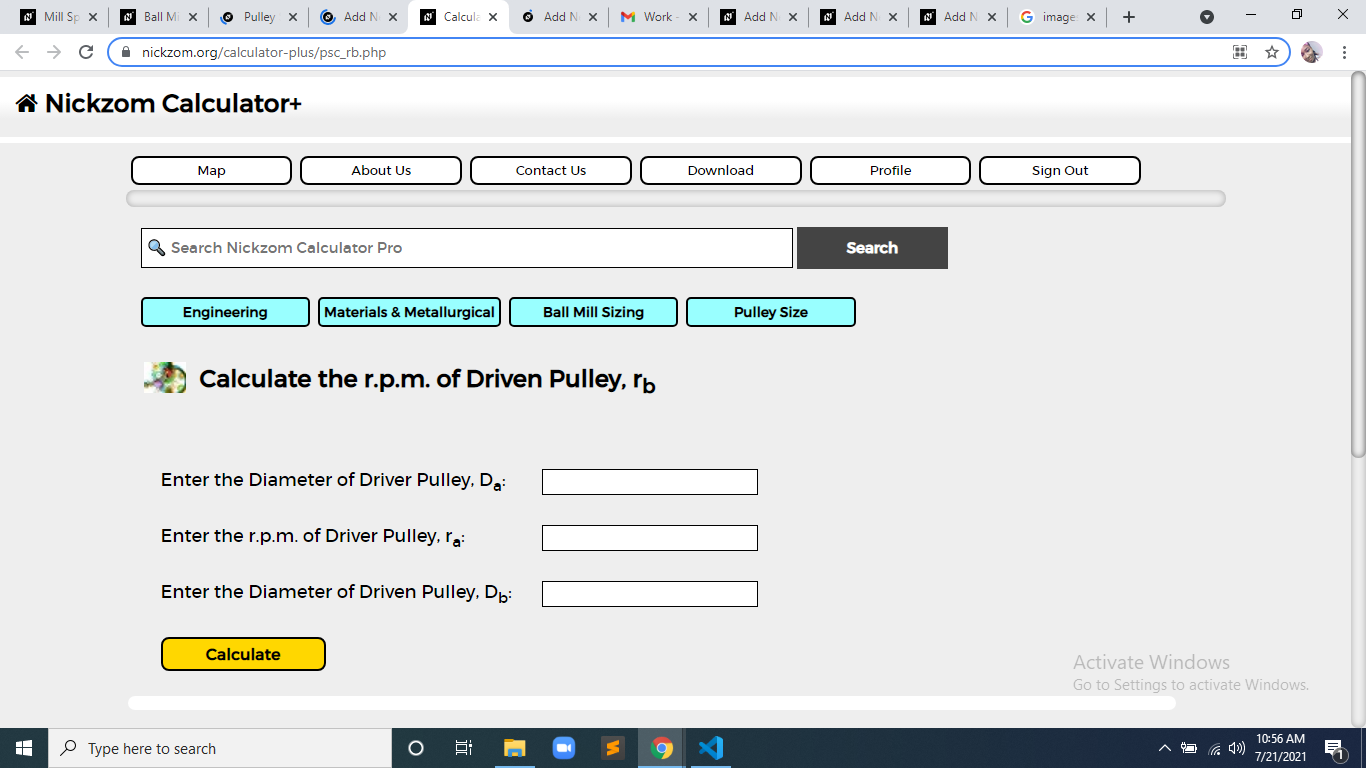Now, enter the value appropriately and accordingly for the parameter as required by the Diameter of Driver Pulley (Da) is 10, r.p.m. of Driver Pulley (rais 4 and Diameter of Driven Pulley (Db) is 6.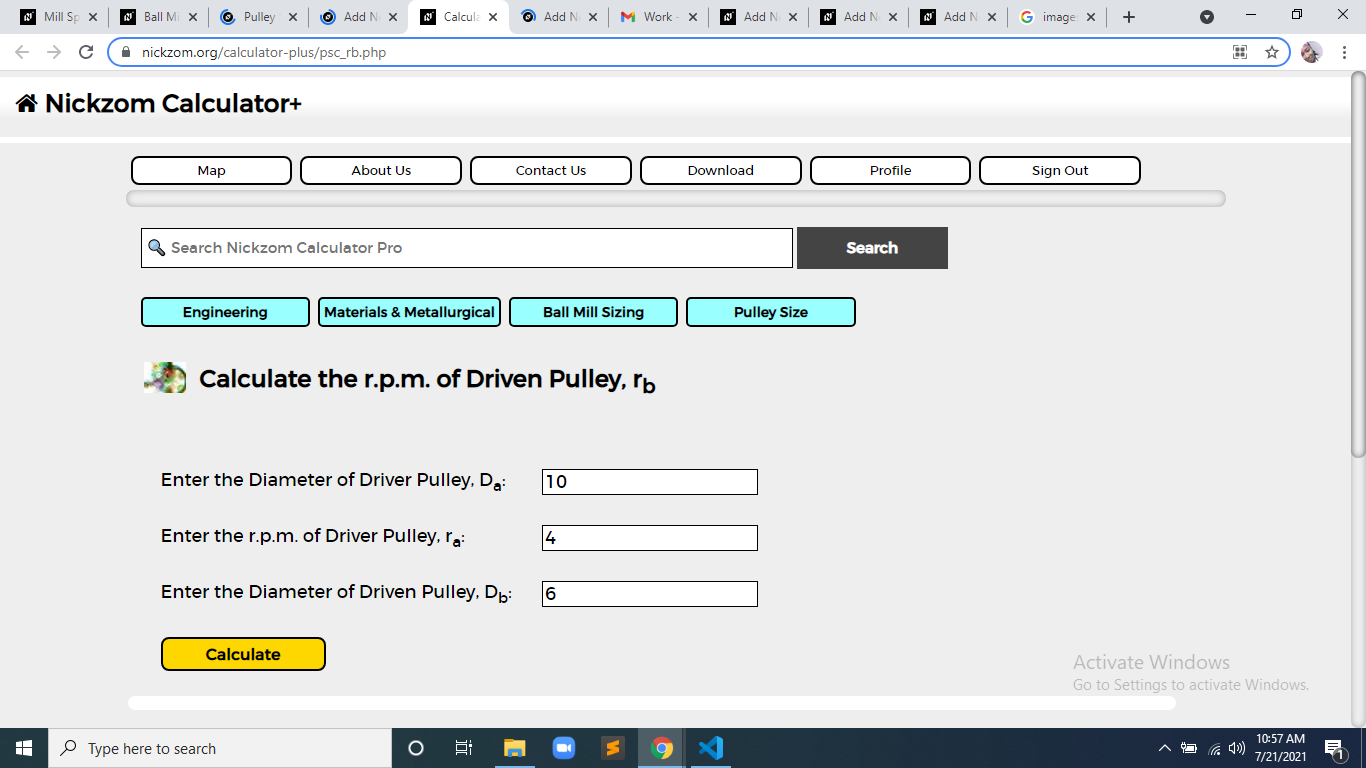Finally, Click on Calculate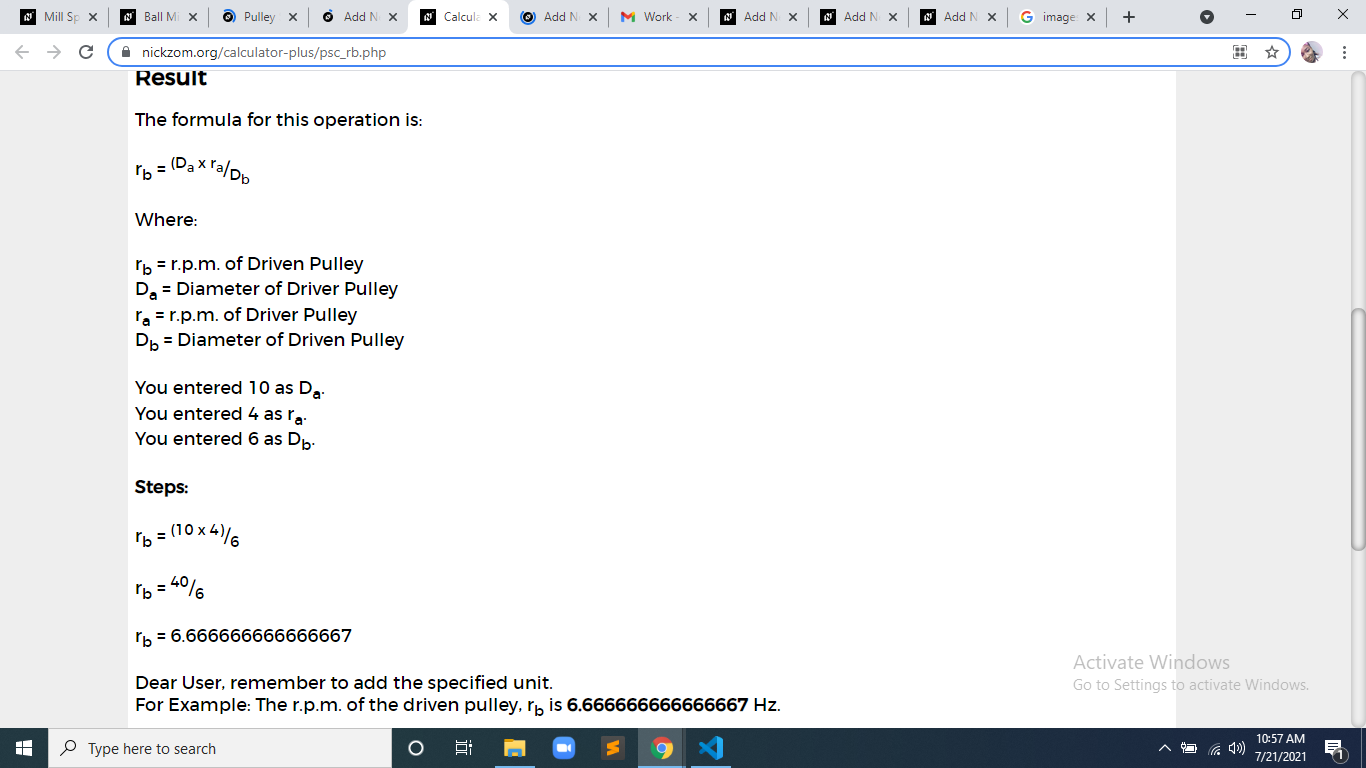As you can see from the screenshot above, Nickzom Calculator– The Calculator Encyclopedia solves for the r.p.m of driven pulley and presents the formula, workings and steps too.